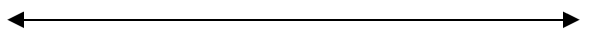# Rational vs. Irrational numbers

### Rational vs. Irrational numbers

Sometimes, it's hard to tell the difference between rational and irrational numbers. Rational numbers can be written as "fraction", "repeating decimals" or "terminating decimal". Irrational numbers are the opposite of that. In this session, we will learn how to identify rational and irrational numbers.

#### Lessons

• Introduction
a)
Rational numbers VS. Irrational numbers
• What are rational numbers?
• What are irrational numbers?
• How are they different from each other?

• 1.
Identify rational and irrational numbers.
1.45667
$\sqrt{7}$
3.56565656…
-2.393399333999…

• 2.
Order the following numbers on the number line.
a)
$\sqrt{13}$
$\pi$
$\sqrt{60}$
3$\sqrt{25}$
-$\sqrt{0.20}$